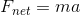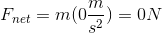# GED Science : Newton's Laws

## Example Questions

### Example Question #1 : Physics

Which of the following best illustrates Newton's Second Law?

A ball is rolled across a table and does not stop until it hits an area with friction

If an object is not accelerating, then the net force on it is zero

As a hammer hits a nail, the nail also exerts a force on the hammer

When two cymbals collide, some kinetic energy is converted into sound

If an object is not accelerating, then the net force on it is zero

Explanation:

Newton's Second Law is best represented as an equation, in which the product of mass and acceleration is equal to net force:By this principle, if there is zero acceleration, then the force must also be zero.A non-zero acceleration must be present if there is a non-zero net force.

### Example Question #1 : Physics

Which of the following is the best example of Newton's Third Law?

A spinning top will not fall unless there is friction with the surface on which it spins

If an object's weight rests on the floor, then the floor will exert a normal force on the object

When two objects collide, the total initial and total final momentum will be equal

The force of being hit by an object is determined by its mass and acceleration